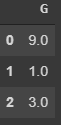Search

# The Pandas fillna and dropna Methods

Let's say that you are trying to learn something new in NumPY and Pandas called the fillna and dropna methods (obviously for the convenience of this blog), but you don't know where to start. Well then this blog that you are reading right now is here to help! Now if you have any questions about the subject of this blog, read on to the QUESTIONS section and if you don't have any questions, you are free to skip to the next section. Now let's crack on with the next part!

QUESTIONS:

1. What is the fillna method?

2. What is the dropna method?

3. Why are the fillna and dropna methods used?

In many cases, you will want to replace missing values in a Pandas DataFrame instead of dropping it completely. The fillna method is designed for this.

Pandas has a built-in method called dropna. When applied against a DataFrame, the dropna method will remove any rows that contain a NaN value.

In many cases, you will want to replace missing values in a Pandas DataFrame instead of dropping it completely. The fillna method is designed for this. Pandas has a built-in method called dropna. When applied against a DataFrame, the dropna method will remove any rows that contain a NaN value. This is what the dropna method does.

Now after that brief explanation of some things in this blog, we will now move on with the coding section.

CODING:

Now before we start, if you want to follow along with me, you can use Kaggle or Google Colaboratory.

CODING: THE FILLNA SECTION:

1. Always Import, Or Else(Import the libraries)...

First, we will have to import all needed libraries(as always).

```import numpy as np
import pandas as pd```

2. Variables, Variables, and NaN(Defining Variables and NaN(Not a Number))

If you tried to print a table without defining some variables, you would definitely

get some errors. So let's define some variables! The code is down below:

```df = pd.DataFrame(np.array([[1, 6, 2],[2, np.nan, 1],[np.nan, np.nan, 9]]))

df.columns = ['Q', 'Z', 'H']

df```

The output for this is down below:3. NaN is a Bit Boring.... Let's Change it Using the fillna Method!(The fillna Method)

Did you see what the heading above said? The "fillna Method"? Well, this step will tell you how you change NaN into a random word, letter, sentence, or emoji. The code is down below (the blank represents anything):

`df.fillna('             ')`

This is what I chose to replace NaN with:

`df.fillna('😎')`

The output is down below:4. Some Really Confusing and Complicated Stuff....

Just kidding! This step is about reading the first step of the dropna method so... what are you waiting for! Let's go to the first step of the dropna section!

CODING: THE DROPNA SECTION:

1.Always Import, Or Else(Import the libraries)...

First, we will have to import all needed libraries(as always).

```import numpy as np
import pandas as pd```

2. Variables, Variables, and Some Other Stuff(Defining Variables)

If you tried to print a table without defining some variables, you would definitely

get some errors. So let's define some variables! The code is down below:

```df1 = pd.DataFrame(np.array([[9, 2, 7],[1, np.nan, np.nan],[3, np.nan, 6]]))

df1.columns = ['G', 'P', 'I']

df1```

The output for this is down below:3. Let's Drop a Few Things With the dropna Method(The dropna Method)!

Now, let's drop a few things with the dropna method. But how do you do this? The code is down below:

`df1.dropna()`

The output is down below:Another way to drop some things with the dropna method is like this:

`df1.dropna(axis=1)`

The output is down below:CONCLUSION:

And with that, this blog will end. I hope you like this blog and without further ado, happy reading!

See All